# H-principle

Jump to: navigation, search

homotopy principle

A term having its origin in papers by M. Gromov in the 1960s and 1970s, some in collaboration with Y. Eliashberg and V. Rokhlin. It applies to partial differential equations or inequalities which have, very roughly speaking, as many solutions as predicted by topology.

The foundational example is the immersion theorem of S. Smale and M. Hirsch, which states the following: Letandbe smooth manifolds without boundary and suppose that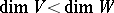or thatis non-compact. Then a smooth mapping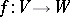is homotopic (cf. also Homotopy) to a smooth immersion if and only if it can be covered by a continuous bundle mapping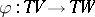which is injective on each fibre. The-principle provides a general language to formulate this and other geometric problems.

Letbe a smooth fibration and letthe space of-jets of smooth sections of. A section of the bundleis called holonomic if it is the-jet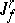of a sectionof. A differential relation (of order) imposed on sections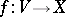is a subset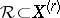. On says thatsatisfies the-principle if every section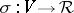is homotopic to a holonomic sectionthrough a homotopy of sections.

There are several versions of the-principle (relative, with parameters, etc.). For instance, the-principle is called dense if a section of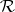can be homotoped into a holonomic section by a homotopy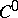-close to the original section.

To formulate the immersion theorem above in this language, one takes the trivial fibrationand defines the immersion relation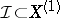by stipulating that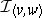consist of the injective linear mappings in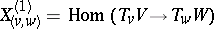. The immersion theorem then says that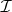satisfies the-principle.

A surprising number of geometrically significant relations satisfy the-principle, and Gromov has developed powerful methods for proving the-principle. The fundamental reference for this subject is [a1]. The principal methods for proving the-principle are removal of singularities, continuous sheaves, and convex integration.

The simplest instance of relations satisfying the-principle is arguably that of open relations over open manifolds (or in the case of extra dimension), subject to some naturality conditions. A very readable account of this theory can be found in [a2]. One particular application of this form of the-principle is to symplectic and contact geometry: If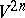is open, then every non-degenerate-form(i.e. satisfying) is homotopic to an exact non-degenerate-form, i.e. an exact symplectic form. Similarly, if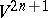is open and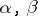are a-form, respectively a-form, with, thenis homotopic to a contact form, that is,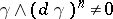. Other important applications of the-principle are the Nash–Kuiper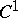-isometric immersion theorem and the classification of isotropic immersions (see Isotropic submanifold; Contact surgery) in contact geometry.